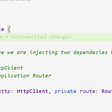# Data Structure: Bubble Sort?

What is a bubble sort technique?

Not the most efficient technique in terms of time complexity, but hey it can be optimized. The bubble sort technique, in a nutshell, is taking an array of adjacent items and comparing one to the next. If for any reason the items do not go from increasing order the element will be swapped.

How does it work

We can take an example array of [4,6,1,7,3,2,5]. We can start by iterating through the array.

`for(let i = array.length - 1; i > 0; i--)`

Notice how we are starting to decrementing instead of incrementing the array. This is because we want to decrement each time we go through an item.

Once the first iteration is established we can begin our second set of iteration. We won’t need to decrement. We can increment through each item like this.

`for(let j = 0; j < array.length; j++)`

Now it’s time to set our conditional statement. Our main purpose is to swap if the current item is greater than the next.

`if(array[j] > array[j + 1])`

Furthermore, we swap our items. And return the array. Here is a full solution below.

`function bubbleSort(array){    for(let i = array.length - 1; i > 0; i--){        for(let j = 0; j < array.length; j++){            if(array[j] > array[j + 1]){                //swap and return array                let temp = array[j]                array[j] = array[j + 1]                array[j + 1] = temp            }        }    }return array}//result [1, 2, 3, 4, 5, 6, 7]`

What is the time complexity?

Given the fact we had to iterate twice the time complexity for this technique runs an O(n)² making it the worst-case scenario. However, it can be optimized.

--

--

--

## More from Woodelin florveus

Love podcasts or audiobooks? Learn on the go with our new app.

## Top Ten JavaScript Interview Questions and Answers for beginners## CRUD application using NestJS and MongoDB## JavaScript Interview Questions (Part 2)## Why You Should Learn JavaScript First?## Angular: Understanding Life cycle hooks/events## YouTube Video | YDKJS — Scopes and Closures — 1## Utilizing StackOverflow to Code Efficiently## Liskov Substitution Principle